# Matchstick Puzzles for Kids - MyHomeSchoolMath

myhomeschoolmath.comTitle
Go to content## Matchstick Puzzles for Kids

Matchstick Puzzles
Matchstick puzzles that are set like math problems or shapes and can be solved by logical reasoning. Solve matchstick puzzles by moving, adding and removing matches until you find the right solution. Have fun!

Can you get this equation right without moving a single matchstick?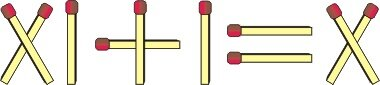Turn just one matchstick to get the equation right (without changing the matchstick position).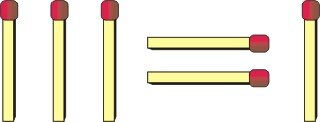Giraffe
Move just one matchstick so the giraffe faces the other way.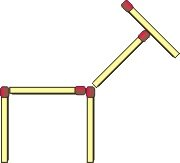Move two matches so that the red ball is no longer on the shovel but next to the shovel.Move three matches to make four squares, all the same size - the same size as the original squares.Rearrange two matches to make four squares, all the same size - the same size as the original squares.Rearrange four matches to make two smaller arrows.Move two matchsticks to make four triangles that are all the same size.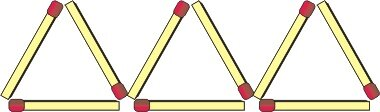Move three matchsticks to make five triangles.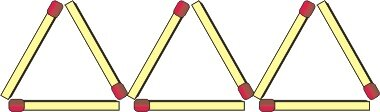Take away three matches to make three squares, all the same size - the same size as the original squares.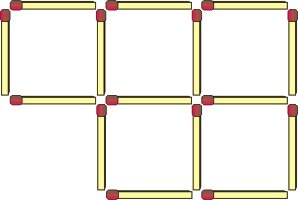A simple matchstick problem: 13 one equals ten.Move two matchsticks to make five squares, all of the same size.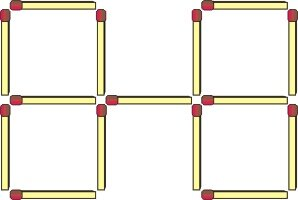Move four matches to make ten squares.Move two matchsticks so that the equation is correct.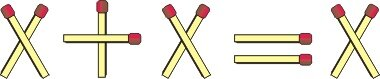Move only one match to make a correct equation.Move two matchsticks to make two squares.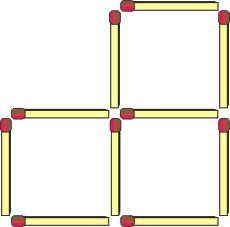Flip two matches to make the fish face the other direction.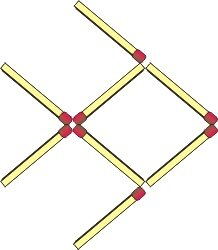Move two matches to make the cow face the opposite direction (to the left).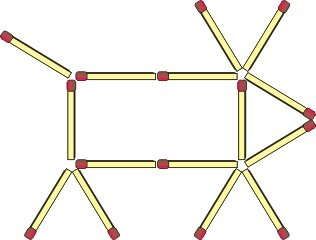Move three matches to make the fish look to the left.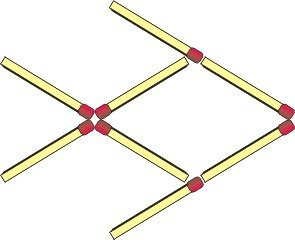Move two matchsticks to make the house face the opposite direction.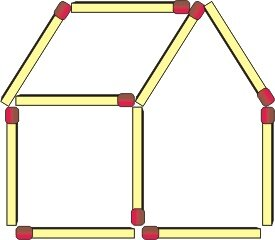A simple matchstick problem: 6 ones equals thirty.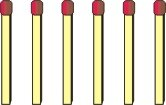Rearrange two matchsticks to make two triangles.Move two matchsticks to make the largest possible three-digit number.You can move only one match to get four triangles.Move only one match to make a square.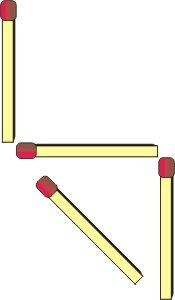The chair fell over. Move two matches so it comes back to a standing position.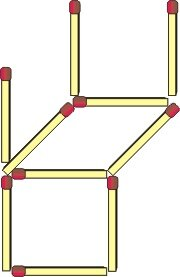The chair fell over. Flip three matches so it comes back to a standing position.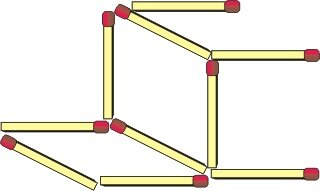Matchstick Puzzles in the form of a mathematical equation

Back to content